# Selina solutions for Concise Mathematics Class 7 ICSE chapter 20 - Mensuration [Latest edition]

#### Chapters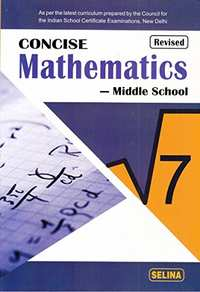## Chapter 20: Mensuration

Exercise 20 (A)Exercise 20 (B)
Exercise 20 (A)

### Selina solutions for Concise Mathematics Class 7 ICSE Chapter 20 MensurationExercise 20 (A)

Exercise 20 (A) | Q 1

The length and the breadth of a rectangular plot are 135 m and 65 m. Find, its perimeter and the cost of fencing it at the rate of ₹60 per m.

Exercise 20 (A) | Q 2

The length and breadth of a rectangular field are in the ratio 7 : 4. If its perimeter is 440 m, find its length and breadth. Also, find the cost of fencing it @ ₹150 per m.

Exercise 20 (A) | Q 3

The length of a rectangular field is 30 m and its diagonal is 34 m. Find the breadth of the field and its perimeter.

Exercise 20 (A) | Q 4

The diagonal of a square is 12sqrt2 cm. Find its perimeter.

Exercise 20 (A) | Q 5

Find the perimeter of a rectangle whose length = 22.5 m and breadth = 16 dm.

Exercise 20 (A) | Q 6

Find the perimeter of a rectangle with length = 24 cm and diagonal = 25 cm

Exercise 20 (A) | Q 7

The length and breadth of the rectangular piece of land area in the ratio of 5 : 3. If the total cost of fencing it at the rate of ₹48 per metre is ₹19,200, find its length and breadth.

Exercise 20 (A) | Q 8

A wire is in the shape of square of side 20 cm. If the wire is bent into a rectangle of length 24 cm, find its breadth.

Exercise 20 (A) | Q 9.1

If P = perimeter of a rectangle, l= its length and b = its breadth find P, if l = 38 cm and b = 27 cm

Exercise 20 (A) | Q 9.2

If P = perimeter of a rectangle, l= its length and b = its breadth find b, if P = 88 cm and l = 24 cm

Exercise 20 (A) | Q 9.3

If P = perimeter of a rectangle, l= its length and b = its breadth find b, if l, if P = 96 m and b = 28 m

Exercise 20 (A) | Q 10

The cost of fencing a square field at the rate of the cost of fencing 440 m = ₹150 × 440 = ₹75 per meter is the cost of fencing 440 m = ₹150 × 440 = ₹67,500. Find the perimeter and the side of the square field.

Exercise 20 (A) | Q 11

The length and the breadth of a rectangle are 36 cm and 28 cm. If its perimeter is equal to the perimeter of a square, find the side of the square.

Exercise 20 (A) | Q 12

The radius of a circle is 21 cm. Find the circumference (Take π = 3 1/7).

Exercise 20 (A) | Q 13

The circumference of a circle is 440 cm. Find its radius and diameter. (Take π = 22/7)

Exercise 20 (A) | Q 14

The diameter of a circular field is 56 m. Find its circumference and cost of fencing it at the rate of ₹80 per m. (Take π =22/7)

Exercise 20 (A) | Q 15

The radius of two circles are 20 cm and 13 cm. Find the difference between their circumferences. (Take π =22/7)

Exercise 20 (A) | Q 16

The diameter of a circle is 42 cm, find its perimeter. If the perimeter of the circle is doubled, what will be the radius of the new circle? (Take π = 22/7)

Exercise 20 (A) | Q 17

The perimeter of a square and the circumference of a circle are equal. If the length of each side of the square is 22 cm, find:
(i) perimeter of the square.
(ii) circumference of the circle.

Exercise 20 (A) | Q 18

Find the radius of the circle whose circumference is equal to the sum of the circumferences of the circles having radius 15 cm and 8 cm.

Exercise 20 (A) | Q 19

Find the diameter of a circle whose circumference is equal to the sum of circumference of circles with radius 10 cm, 12 cm, and 18 cm.

Exercise 20 (A) | Q 20

The circumference of a circle is eigth time the circumference of the circle with radius 12 cm. Find its diameter.

Exercise 20 (A) | Q 21

The radius of two circles are in the ratio 3: 5, find the ratio between their circumferences.

Exercise 20 (A) | Q 22

The circumferences of two circles are in the ratio 5: 7, find the ratio between their radius.

Exercise 20 (A) | Q 23

The perimeters of two squares are in the ratio 8:15, find the ratio between the lengths of their sides.

Exercise 20 (A) | Q 24

The lengths of the sides of two squares are in the ratio 8:15, find the ratio between their perimeters.

Exercise 20 (A) | Q 25

Each side of a square is 44 cm. Find its perimeter. If this perimeter is equal to the circumference of a circle, find the radius of the circle.

Exercise 20 (B)

### Selina solutions for Concise Mathematics Class 7 ICSE Chapter 20 MensurationExercise 20 (B)

Exercise 20 (B) | Q 1

Find the area of a rectangle whose length and breadth are 25 m and 16 cm.

Exercise 20 (B) | Q 2

The diagonal of a rectangular board is 1 m and its length is 96 cm. Find the area of the board.

Exercise 20 (B) | Q 3

The sides of a rectangular park are in the ratio 4 : 3. If its area is 1728 m2, find
(i) its perimeter
(ii) cost of fencing it at the rate of ₹40 per meter.

Exercise 20 (B) | Q 4

A floor is 40 m long and 15 m broad. It is covered with tiles, each measuring 60 cm by 50 cm. Find the number of tiles required to cover the floor.

Exercise 20 (B) | Q 5

The length and breadth of a rectangular piece of land are in the ratio 5 : 3. If the total cost of fencing it at the rate of ₹24 per meter is ₹9600, find its :
(ii) area
(iii) cost of levelling at the rate of ₹60 per m2.

Exercise 20 (B) | Q 6

Find the area of the square whose perimeter is 56 cm.

Exercise 20 (B) | Q 7

A square lawn is surrounded by a path 2.5 m wide. If the area of the path is 165 m2 find the area of the lawn.

Exercise 20 (B) | Q 8.1

In the figure given below, find the area of shaded region: (All measurements are in cm)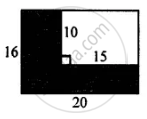Exercise 20 (B) | Q 8.2

In the figure given below, find the area of shaded region: (All measurements are in cm)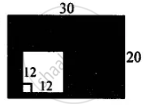Exercise 20 (B) | Q 8.3

In the figure given below, find the area of shaded region: (All measurements are in cm)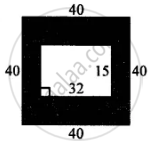Exercise 20 (B) | Q 8.4

In the figure given below, find the area of shaded region: (All measurements are in cm)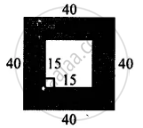Exercise 20 (B) | Q 8.5

In the figure given below, find the area of shaded region: (All measurements are in cm)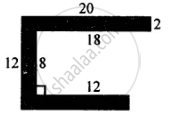Exercise 20 (B) | Q 9

One side of a parallelogram is 20 cm and its distance from the opposite side is 16 cm. Find the area of the parallelogram.

Exercise 20 (B) | Q 10

The base of a parallelogram is thrice it height. If its area is 768 cm2, find the base and the height of the parallelogram.

Exercise 20 (B) | Q 11

Find the area of the rhombus, if its diagonals are 30 cm and 24 cm.

Exercise 20 (B) | Q 12

If the area of a rhombus is 112 cm2 and one of its diagonals is 14 cm, find its other diagonal.

Exercise 20 (B) | Q 13

One side of a parallelogram is 18 cm and its area is 153 cm2. Find the distance of the given side from its opposite side.

Exercise 20 (B) | Q 14

The adjacent sides of a parallelogram are 15 cm and 10 cm. If the distance between the longer sides is 6 cm, find the distance between the shorter sides.

Exercise 20 (B) | Q 15

The area of a rhombus is 84 cm2 and its perimeter is 56 cm. Find its height.

Exercise 20 (B) | Q 16

Find the area of a triangle whose base is 30 cm and the height is 18 cm.

Exercise 20 (B) | Q 17

Find the height of a triangle whose base is 18 cm and the area is 270 cm2.

Exercise 20 (B) | Q 18

The area of a right-angled triangle is 160 cm2. If its one leg is 16 cm long, find the length of the other leg.

Exercise 20 (B) | Q 19

Find the area of a right-angled triangle whose hypotenuse is 13 cm long and one of its legs is 12 cm long.

Exercise 20 (B) | Q 20

Find the area of an equilateral triangle whose each side is 16 cm. (Take sqrt3= 1.73)

Exercise 20 (B) | Q 21

The sides of a triangle are 21 cm, 17 cm, and 10 cm. Find its area.

Exercise 20 (B) | Q 22

Find the area of an isosceles triangle whose base is 16 cm and the length of each of the equal sides is 10 cm.

Exercise 20 (B) | Q 23

Find the base of a triangle whose area is 360 cm2 and height is 24 cm.

Exercise 20 (B) | Q 24

The legs of a right-angled triangle are in the ratio 4 : 3 and its area is 4056 cm2. Find the length of its legs.

Exercise 20 (B) | Q 25

The area of an equilateral triangle is (64xxsqrt3) cm2. Find the length of each side of the triangle.

Exercise 20 (B) | Q 26

The sides of a triangle are in the ratio 15 : 13 : 14 and its perimeter is 168 cm. Find the area of the triangle.

Exercise 20 (B) | Q 27

The diameter of a circle is 20 cm. Taking π = 3.14, find the circumference and its area.

Exercise 20 (B) | Q 28

The circumference of a circle exceeds its diameter by 18 cm. find the radius of the circle.

Exercise 20 (B) | Q 29

The ratio between the radius of two circles is 5 : 7. Find the ratio between their:
(i) circumference
(ii) areas

Exercise 20 (B) | Q 30

The ratio between the areas of two circles is 16 : 9. Find the ratio between their :
(ii) diameters
(iii) circumference

Exercise 20 (B) | Q 31

A circular racing track has inner circumference 528 m and outer circumference 616 m. Find the width of the track.

Exercise 20 (B) | Q 32

The inner circumference of a circular track is 264 m and the width of the track is 7 m. Find:
(i) the radius of the inner track.
(ii) the radius of the outer circumference.
(iii) the length of the outer circumference.
(iv) the cost of fencing the outer circumference at the rate of ₹50 per m.

Exercise 20 (B) | Q 33

The diameter of every wheel of a car is 63 cm. How much distance will the car move during the 2000 revolutions of its wheel.

Exercise 20 (B) | Q 34

The diameter of the wheel of a car is 70 cm. How many revolutions will it make to travel one kilometer?

Exercise 20 (B) | Q 35

A metal wire, when bent in the form of a square of largest area, encloses an area of 484 cm2. Find the length of the wire. If the same wire is bent to the largest circle, find:
(i) radius of the circle formed.
(ii) area of the circle.

Exercise 20 (B) | Q 36

A wire is along the boundary of a circle with a radius of 28 cm. If the same wire is bent in the form of a square, find the area of the square formed.

Exercise 20 (B) | Q 37

The length and the breadth of a rectangular paper are 35 cm and 22 cm. Find the area of the largest circle which can be cut out of this paper.

Exercise 20 (B) | Q 38

From each corner of a rectangular paper (30 cm x 20 cm) a quadrant of a circle of radius 7 cm is cut. Find the area of the remaining paper i.e., shaded portion.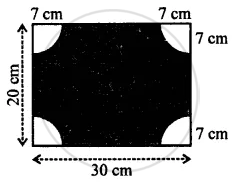## Chapter 20: Mensuration

Exercise 20 (A)Exercise 20 (B)## Selina solutions for Concise Mathematics Class 7 ICSE chapter 20 - Mensuration

Selina solutions for Concise Mathematics Class 7 ICSE chapter 20 (Mensuration) include all questions with solution and detail explanation. This will clear students doubts about any question and improve application skills while preparing for board exams. The detailed, step-by-step solutions will help you understand the concepts better and clear your confusions, if any. Shaalaa.com has the CISCE Concise Mathematics Class 7 ICSE solutions in a manner that help students grasp basic concepts better and faster.

Further, we at Shaalaa.com provide such solutions so that students can prepare for written exams. Selina textbook solutions can be a core help for self-study and acts as a perfect self-help guidance for students.

Concepts covered in Concise Mathematics Class 7 ICSE chapter 20 Mensuration are Concept of Area, Concept of Measurement Using a Basic Unit Area of a Square, Rectangle, Triangle, Parallelogram and Circle, Rings and Combined Figures., Concept of Perimeter, Circumference of a Circle, Mensuration.

Using Selina Class 7 solutions Mensuration exercise by students are an easy way to prepare for the exams, as they involve solutions arranged chapter-wise also page wise. The questions involved in Selina Solutions are important questions that can be asked in the final exam. Maximum students of CISCE Class 7 prefer Selina Textbook Solutions to score more in exam.

Get the free view of chapter 20 Mensuration Class 7 extra questions for Concise Mathematics Class 7 ICSE and can use Shaalaa.com to keep it handy for your exam preparation